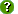# Dataset Details

Data Set ID: primet_229_a_5min_2014
Originator: Christopher Daly
Title: Meteorological data from Primary Met Station at the Andrews Forest. Variables include: fan-aspirated air temperature and four component radiation from 01-Oct-2013 to 01-Oct-2014
Abstract: A three-level hydro-climatological network for data monitoring was established in 1994. The networks at each level are nested to form a coordinated program of data acquisition and measurement. A future vision of linking the benchmark meteorological stations with regional weather stations to expand the future scope of studies was also considered in designing this network. The first-level in this top-down approach consists of Benchmark Meteorological Stations (BMS) and Benchmark Stream Stations. The BMS are designed to represent the environment across the Andrews. These stations are intended to provide complete, long-term, high temporal resolution, meso-scale hydroclimatological data. The location of the BMS network is based on factors such as elevation, aspect, vegetation gradients, and accessibility. Collected meteorological parameters are generally standardized across the BMS as well as methods and instrumentation. Secondary Meteorological Stations also follow standardized methods and serve similar purposes but are somewhat limited in meteorological parameters collected. The Primary Meteorological Station (PRIMET), Central Meteorological Station (CENMET), Upper Lookout Meteorological Station (UPLMET), and Vanilla Leaf Meteorological Station (VANMET) are the four Benchmark Stations, Climatic Station at Watershed 2 (CS2MET) and the Hi-15 Meteorological Station (H15MET) are Secondary Stations.
Key Words: Air temperature, Climate change, Climate data, Meteorological measurements, Monitoring, Photosynthetically active radiation, Precipitation, Relative humidity, Snow accumulation, Snow hydrology, Soil moisture, Soil temperature, Solar radiation, Wind speed, Disturbance
Study Type: Monitoring
Study Period: 01-Oct-2013 to 01-Oct-2014
Bounding Box: West longitude:  unspecified
North latitude:  unspecified
East longitude:  unspecified
South latitude:  unspecified
Site References: PRIMET_229
Downloads:Data Table:  primet_229_a_5min_2014 (Main data table, 105121 records)

Access:  Public

Metadata:  Text (ESA FLED)

Data Files:  Spreadsheet (CSV Text) [20919kb], GCE Data Toolbox File [3728kb], Web Table (HTML text) [657kb]

Column List:

Column Name Units Type Description (show)
1 Site string Site code
2 Date serial number floating-point TmStamp
3 LOGGERID none integer LOGGERID
4 PROGID none floating-point Program ID of program
5 SW_IN_MEAN_600_0_02 W m-2 floating-point Downward shortwave radiation
6 Flag_SW_IN_MEAN_600_0_02 none string QA/QC flags for Downward shortwave radiation (flagging criteria, where "x" is SW_IN_MEAN_600_0_02: x<=-10="I", x>1367="I", x>1060="Q", isnan(x)="M")
7 SW_OUT_MEAN_600_0_02 W m-2 floating-point Upward shortwave radiation
8 Flag_SW_OUT_MEAN_600_0_02 none string QA/QC flags for Upward shortwave radiation (flagging criteria, where "x" is SW_OUT_MEAN_600_0_02: x<=-10="I", x>1367="I", x>1060="Q", isnan(x)="M")
9 LW_IN_MEAN_600_0_02 W m-2 floating-point Corrrected downward longwave radiation (emited from the atmosphere). Correction factor: IR01UpCo=IR01Up+5.67*10^-8*NR01TK^4
10 Flag_LW_IN_MEAN_600_0_02 none string QA/QC flags for Corrrected downward longwave radiation (emited from the atmosphere). Correction factor: IR01UpCo=IR01Up+5.67*10^-8*NR01TK^4 (flagging criteria, where "x" is LW_IN_MEAN_600_0_02: isnan(x)="M")
11 LW_OUT_MEAN_600_0_02 W m-2 floating-point Corrrected upward longwave radiation (emited from the surface). Correction factor: IR01DnCo=IR01Dn+5.67*10^-8*NR01TK^4
12 Flag_LW_OUT_MEAN_600_0_02 none string QA/QC flags for Corrrected upward longwave radiation (emited from the surface). Correction factor: IR01DnCo=IR01Dn+5.67*10^-8*NR01TK^4 (flagging criteria, where "x" is LW_OUT_MEAN_600_0_02: isnan(x)="M")
13 NR_TOT_MEAN_600_0_02 W m-2 floating-point Net radiation total. NetTot=UpTot-DnTot
14 Flag_NR_TOT_MEAN_600_0_02 none string QA/QC flags for Net radiation total. NetTot=UpTot-DnTot (flagging criteria, where "x" is NR_TOT_MEAN_600_0_02: isnan(x)="M")
15 SENSOR_TEMP_600_0_02 deg c floating-point Temperature of sensor body
16 Flag_SENSOR_TEMP_600_0_02 none string QA/QC flags for Temperature of sensor body (flagging criteria, where "x" is SENSOR_TEMP_600_0_02: isnan(x)="M")
17 RAD_IN_AVG W m-2 floating-point Total downward radiation. UpTot=SR01Up+IR01UpCo
18 Flag_RAD_IN_AVG none string QA/QC flags for Total downward radiation. UpTot=SR01Up+IR01UpCo (flagging criteria, where "x" is RAD_IN_AVG: isnan(x)="M")
19 AIRTEMP_MEAN_150_0_06 deg c floating-point Fan aspirated temperature
20 Flag_AIRTEMP_MEAN_150_0_06 none string QA/QC flags for Fan aspirated temperature (flagging criteria, where "x" is AIRTEMP_MEAN_150_0_06: isnan(x)="M", x<=-35="I", x>50="I", x<FOURLO="Q", x>FOURHI="Q", flag_valuechange(x,8,8,1)="Q", flag_valuechange(x,6.5,6.5,1)="V")
21 AIRTEMP_MEAN_250_0_07 deg c floating-point Fan aspirated temperature
22 Flag_AIRTEMP_MEAN_250_0_07 none string QA/QC flags for Fan aspirated temperature (flagging criteria, where "x" is AIRTEMP_MEAN_250_0_07: isnan(x)="M", x<=-35="I", x>50="I", x<FOURLO="Q", x>FOURHI="Q", flag_valuechange(x,8,8,1)="Q", flag_valuechange(x,6.5,6.5,1)="V")
23 AIRTEMP_MEAN_350_0_08 deg c floating-point Fan aspirated temperature
24 Flag_AIRTEMP_MEAN_350_0_08 none string QA/QC flags for Fan aspirated temperature (flagging criteria, where "x" is AIRTEMP_MEAN_350_0_08: isnan(x)="M", x<=-35="I", x>50="I", x<FOURLO="Q", x>FOURHI="Q", flag_valuechange(x,8,8,1)="Q", flag_valuechange(x,6.5,6.5,1)="V")
25 AIRTEMP_MEAN_450_0_09 deg c floating-point Fan aspirated temperature
26 Flag_AIRTEMP_MEAN_450_0_09 none string QA/QC flags for Fan aspirated temperature (flagging criteria, where "x" is AIRTEMP_MEAN_450_0_09: isnan(x)="M", x<=-35="I", x>50="I", x<FOURLO="Q", x>FOURHI="Q", flag_valuechange(x,8,8,1)="Q", flag_valuechange(x,6.5,6.5,1)="V")
27 AIRTEMP_MAX_150_0_06 deg c floating-point Fan aspirated temperature
28 Flag_AIRTEMP_MAX_150_0_06 none string QA/QC flags for Fan aspirated temperature (flagging criteria, where "x" is AIRTEMP_MAX_150_0_06: isnan(x)="M", x<=-35="I", x>50="I", x<FOURLO="Q", x>FOURHI="Q", flag_valuechange(x,8,8,1)="Q", flag_valuechange(x,6.5,6.5,1)="V")
29 AIRTEMP_MAX_250_0_07 deg c floating-point Fan aspirated temperature
30 Flag_AIRTEMP_MAX_250_0_07 none string QA/QC flags for Fan aspirated temperature (flagging criteria, where "x" is AIRTEMP_MAX_250_0_07: isnan(x)="M", x<=-35="I", x>50="I", x<FOURLO="Q", x>FOURHI="Q", flag_valuechange(x,8,8,1)="Q", flag_valuechange(x,6.5,6.5,1)="V")
31 AIRTEMP_MAX_350_0_08 deg c floating-point Fan aspirated temperature
32 Flag_AIRTEMP_MAX_350_0_08 none string QA/QC flags for Fan aspirated temperature (flagging criteria, where "x" is AIRTEMP_MAX_350_0_08: isnan(x)="M", x<=-35="I", x>50="I", x<FOURLO="Q", x>FOURHI="Q", flag_valuechange(x,8,8,1)="Q", flag_valuechange(x,6.5,6.5,1)="V")
33 AIRTEMP_MAX_450_0_09 deg c floating-point Fan aspirated temperature
34 Flag_AIRTEMP_MAX_450_0_09 none string QA/QC flags for Fan aspirated temperature (flagging criteria, where "x" is AIRTEMP_MAX_450_0_09: isnan(x)="M", x<=-35="I", x>50="I", x<FOURLO="Q", x>FOURHI="Q", flag_valuechange(x,8,8,1)="Q", flag_valuechange(x,6.5,6.5,1)="V")
35 AIRTEMP_MIN_150_0_06 deg c floating-point Fan aspirated temperature
36 Flag_AIRTEMP_MIN_150_0_06 none string QA/QC flags for Fan aspirated temperature (flagging criteria, where "x" is AIRTEMP_MIN_150_0_06: isnan(x)="M", x<=-35="I", x>50="I", x<FOURLO="Q", x>FOURHI="Q", flag_valuechange(x,8,8,1)="Q", flag_valuechange(x,6.5,6.5,1)="V")
37 AIRTEMP_MIN_250_0_07 deg c floating-point Fan aspirated temperature
38 Flag_AIRTEMP_MIN_250_0_07 none string QA/QC flags for Fan aspirated temperature (flagging criteria, where "x" is AIRTEMP_MIN_250_0_07: isnan(x)="M", x<=-35="I", x>50="I", x<FOURLO="Q", x>FOURHI="Q", flag_valuechange(x,8,8,1)="Q", flag_valuechange(x,6.5,6.5,1)="V")
39 AIRTEMP_MIN_350_0_08 deg c floating-point Fan aspirated temperature
40 Flag_AIRTEMP_MIN_350_0_08 none string QA/QC flags for Fan aspirated temperature (flagging criteria, where "x" is AIRTEMP_MIN_350_0_08: isnan(x)="M", x<=-35="I", x>50="I", x<FOURLO="Q", x>FOURHI="Q", flag_valuechange(x,8,8,1)="Q", flag_valuechange(x,6.5,6.5,1)="V")
41 AIRTEMP_MIN_450_0_09 deg c floating-point Fan aspirated temperature
42 Flag_AIRTEMP_MIN_450_0_09 none string QA/QC flags for Fan aspirated temperature (flagging criteria, where "x" is AIRTEMP_MIN_450_0_09: isnan(x)="M", x<=-35="I", x>50="I", x<FOURLO="Q", x>FOURHI="Q", flag_valuechange(x,8,8,1)="Q", flag_valuechange(x,6.5,6.5,1)="V")
Last Modified: 06-May-2021 18:22:02